# Telangana SSC Board (TS SSC) Question Paper 1 for Class 10th Maths 2015 In PDF

## Telangana 10th Annual Exam Question Papers 2015 Maths Paper 1 with Solutions – Free Download

Telangana SSC Class 10 Maths 2015 Question Paper 1 with solutions are given by the subject experts at BYJU’S with detailed explanations. The solutions of Telangana 10th Annual Exam Question Papers 2015 Maths Paper 1 are accurate and follow all the guidelines prescribed by the board in terms of marking scheme. Students can also access important concepts of Class 10 Maths, along with the practice questions, so that they can practice them easily at any time. All these question papers of Class 10 Maths and solutions of the same will help the students in clearing their doubts with respect to the approaches to solve the problem correctly.

### Telangana Board SSC Class 10 Maths 2015 Question Paper 1 with Solutions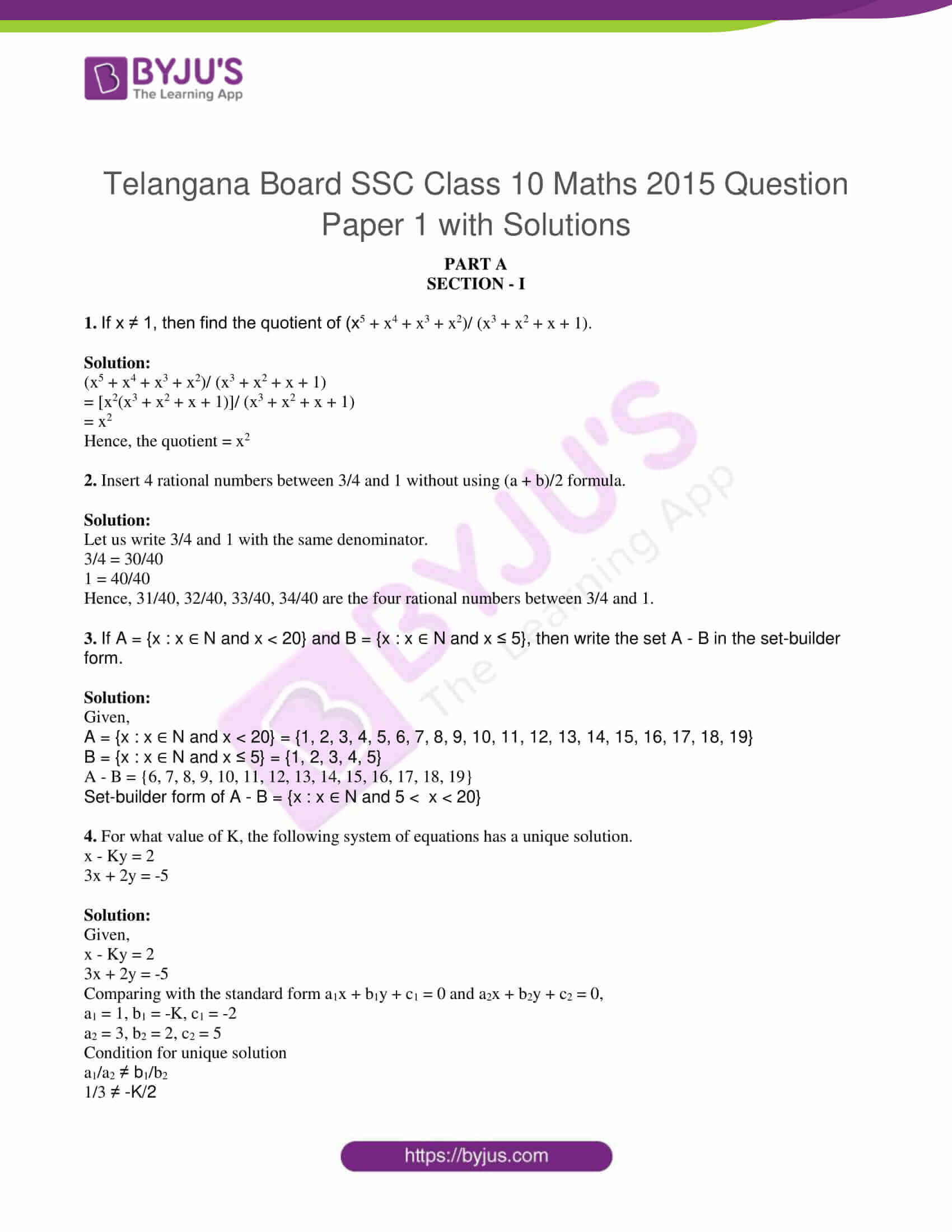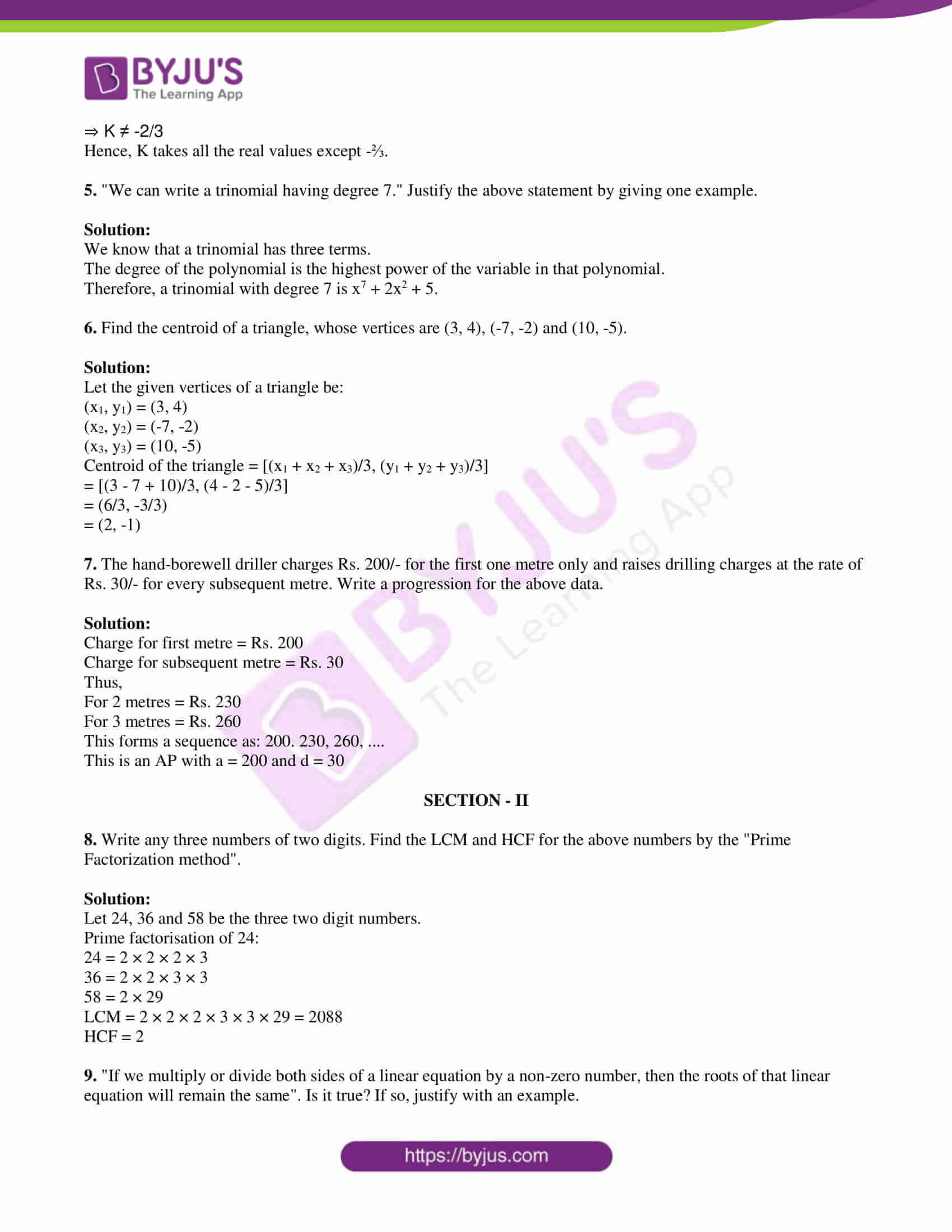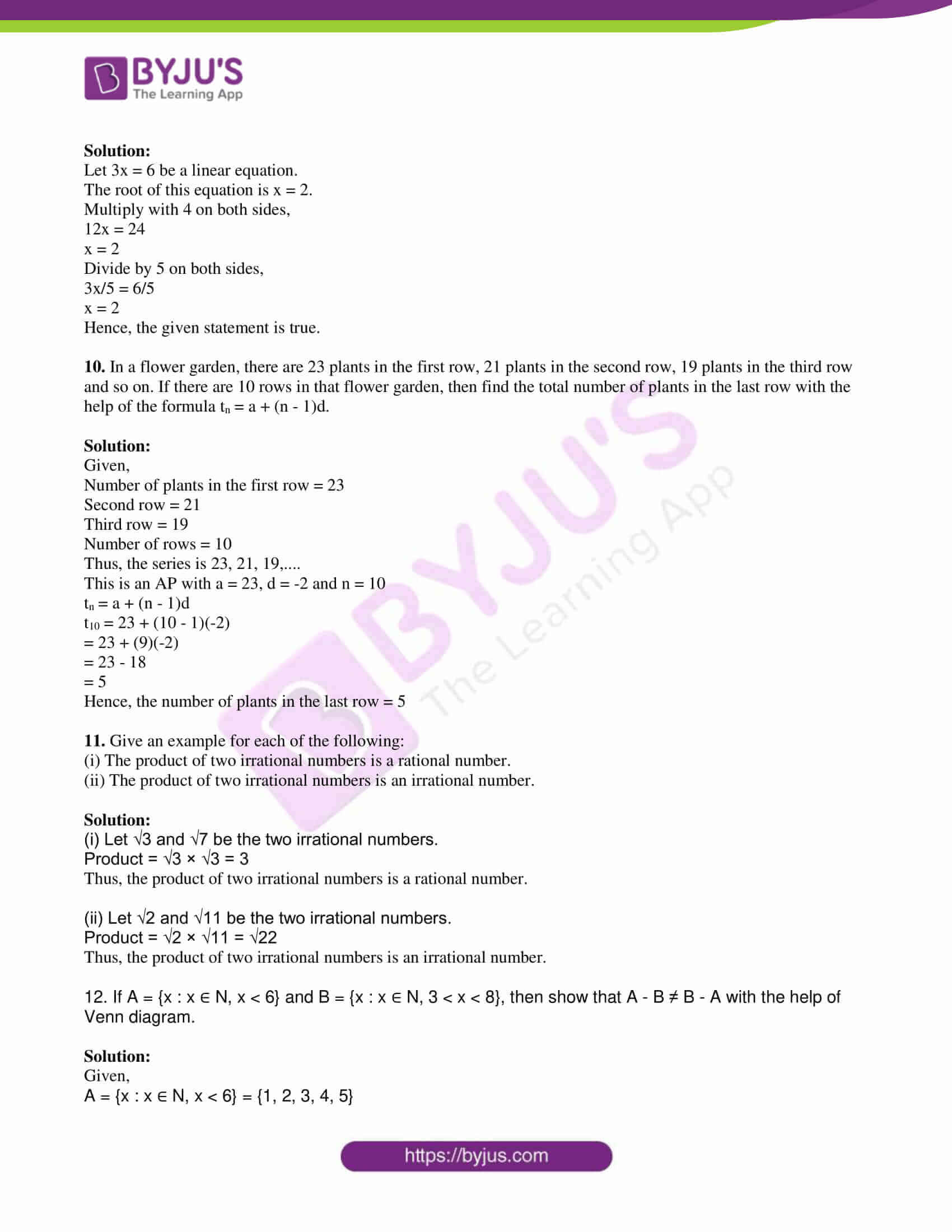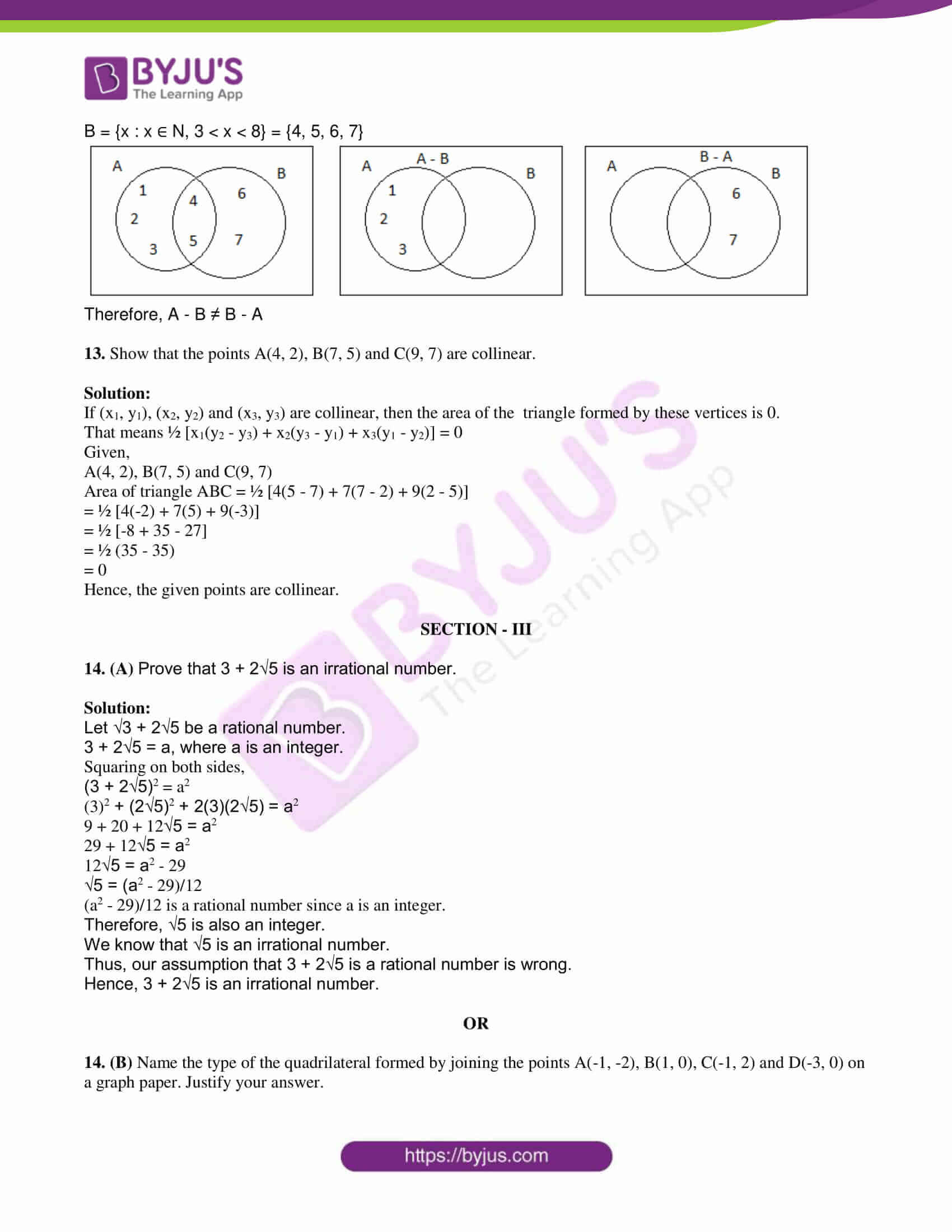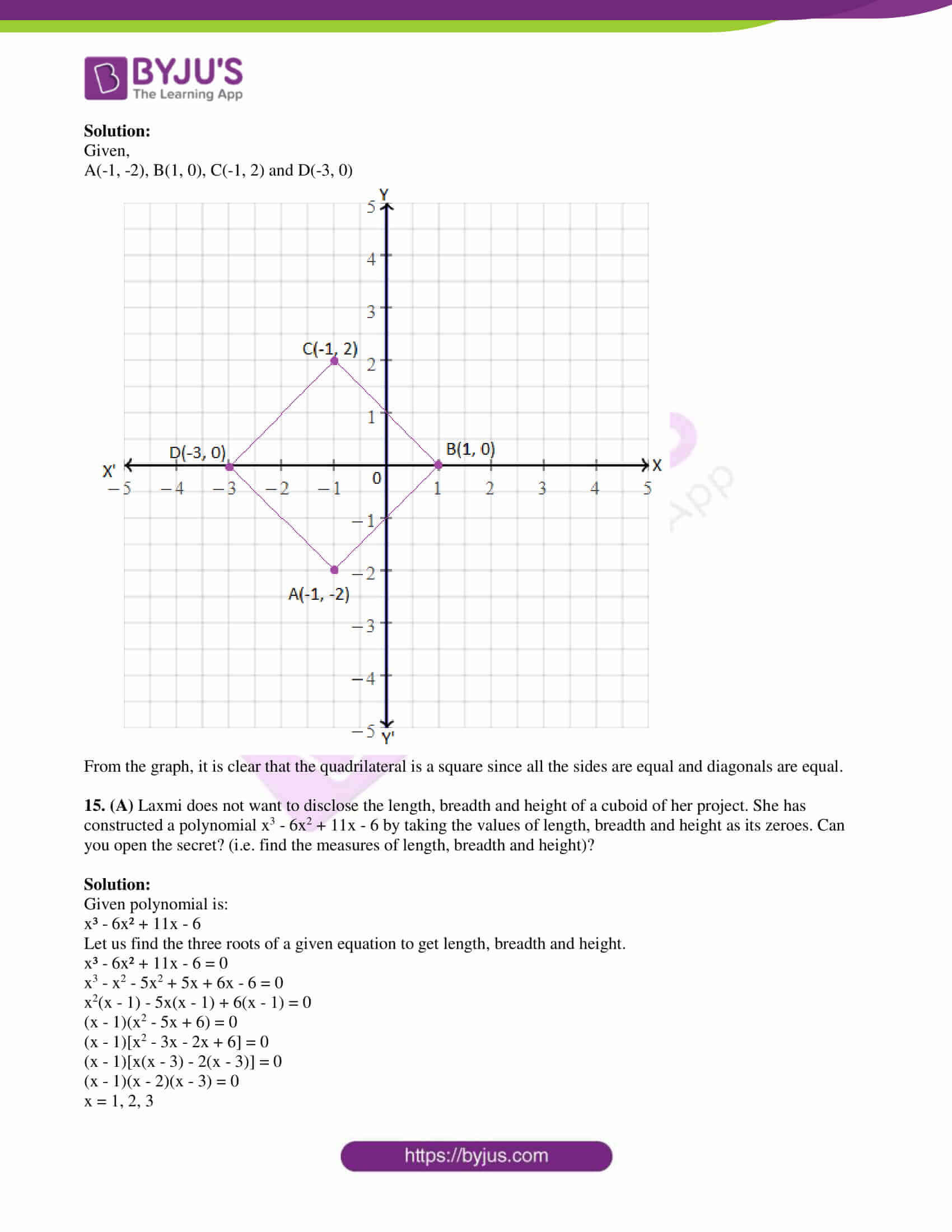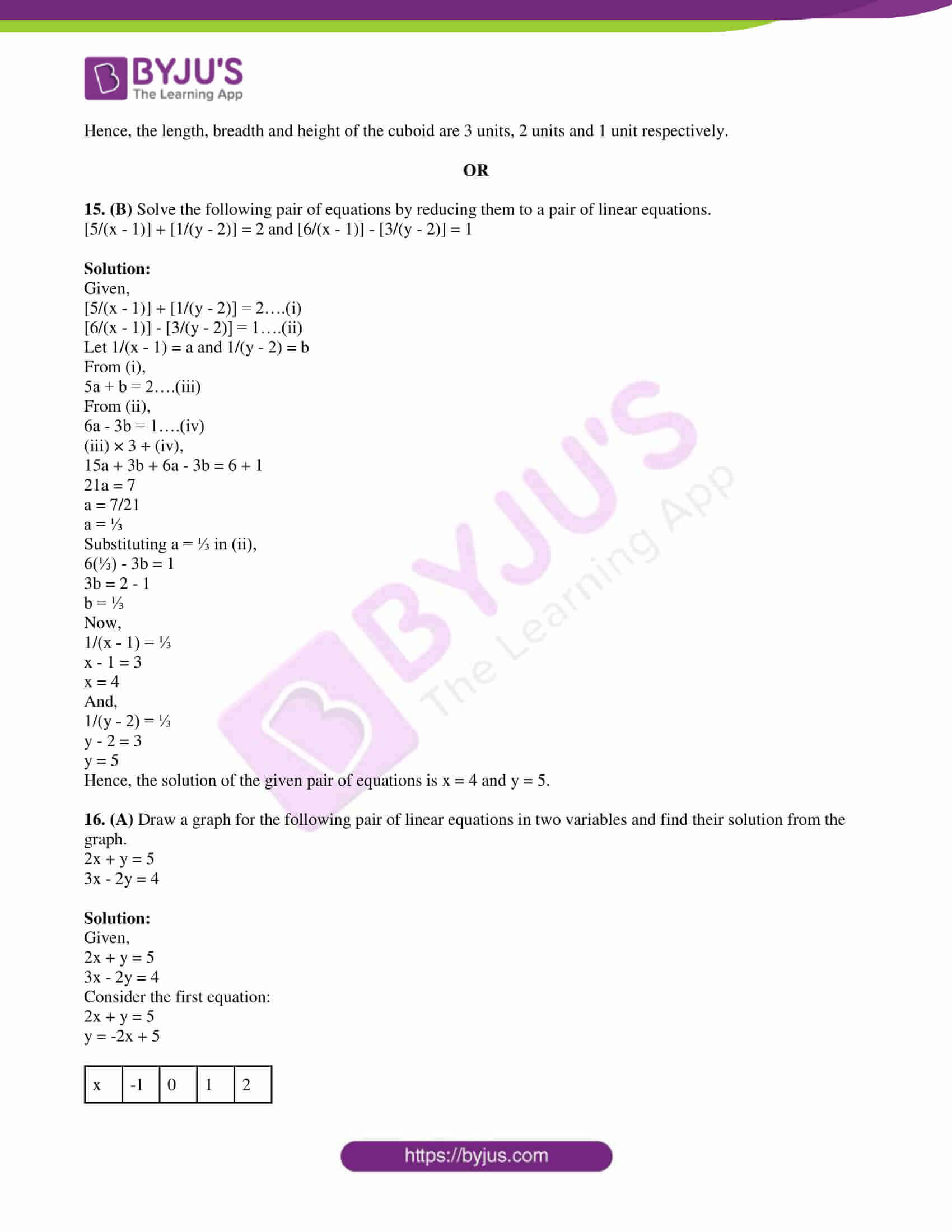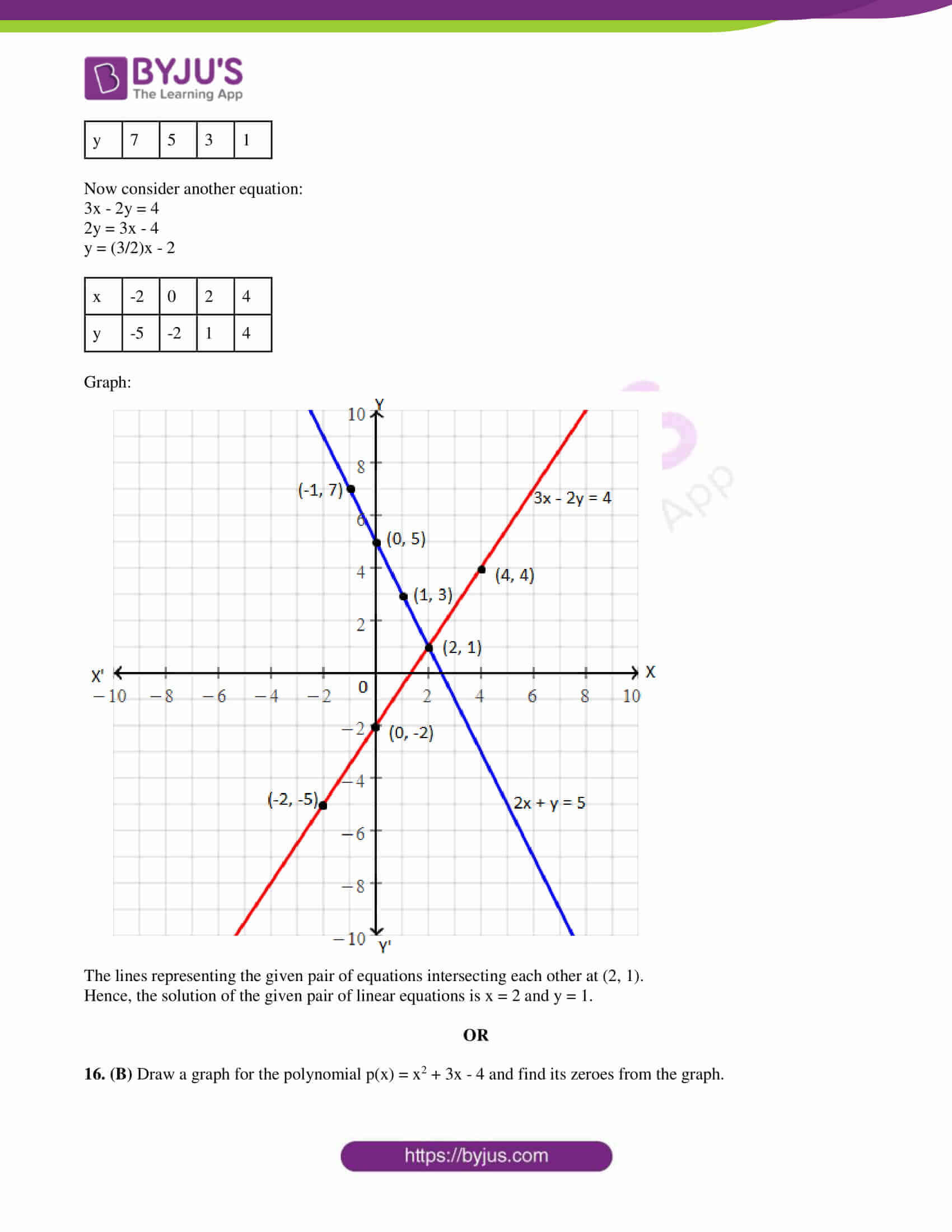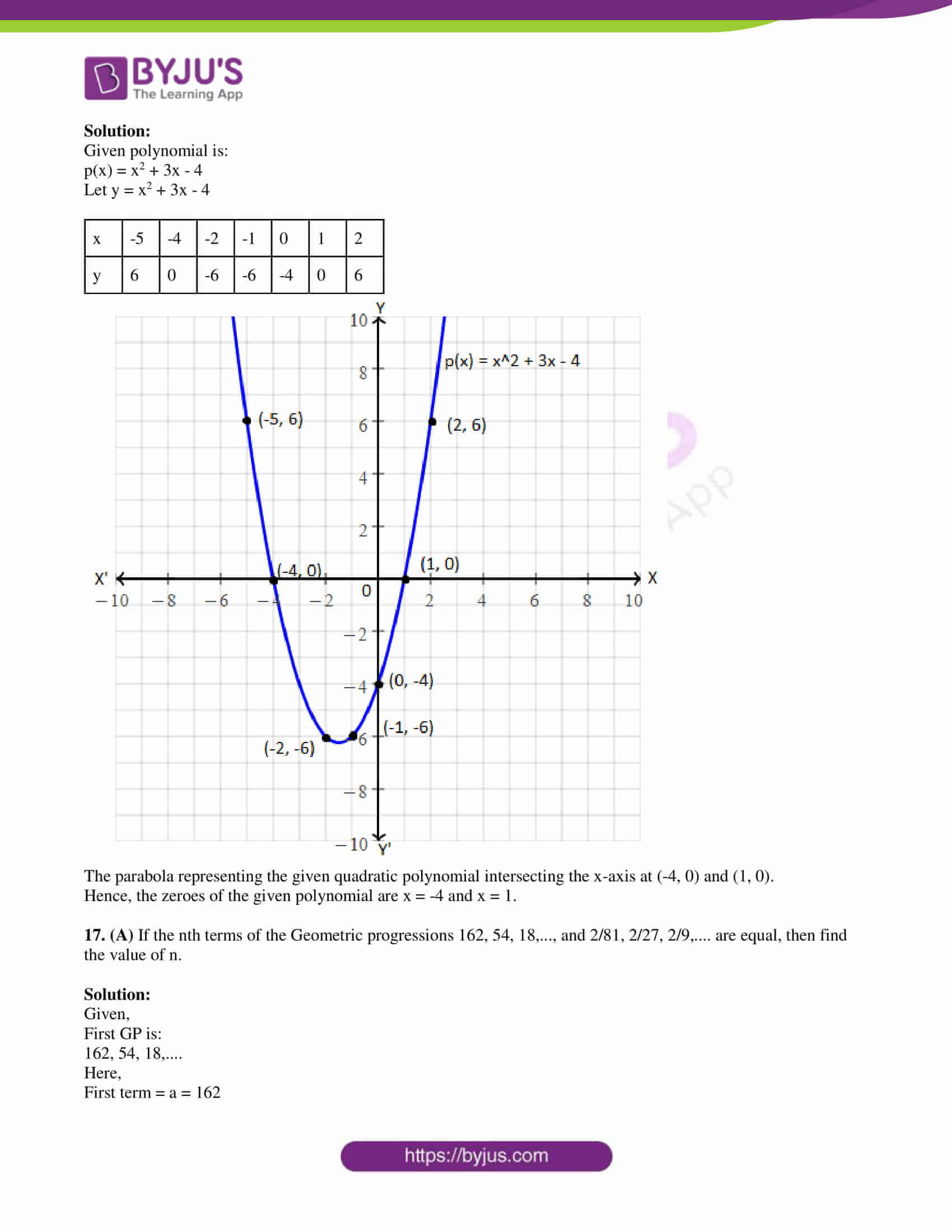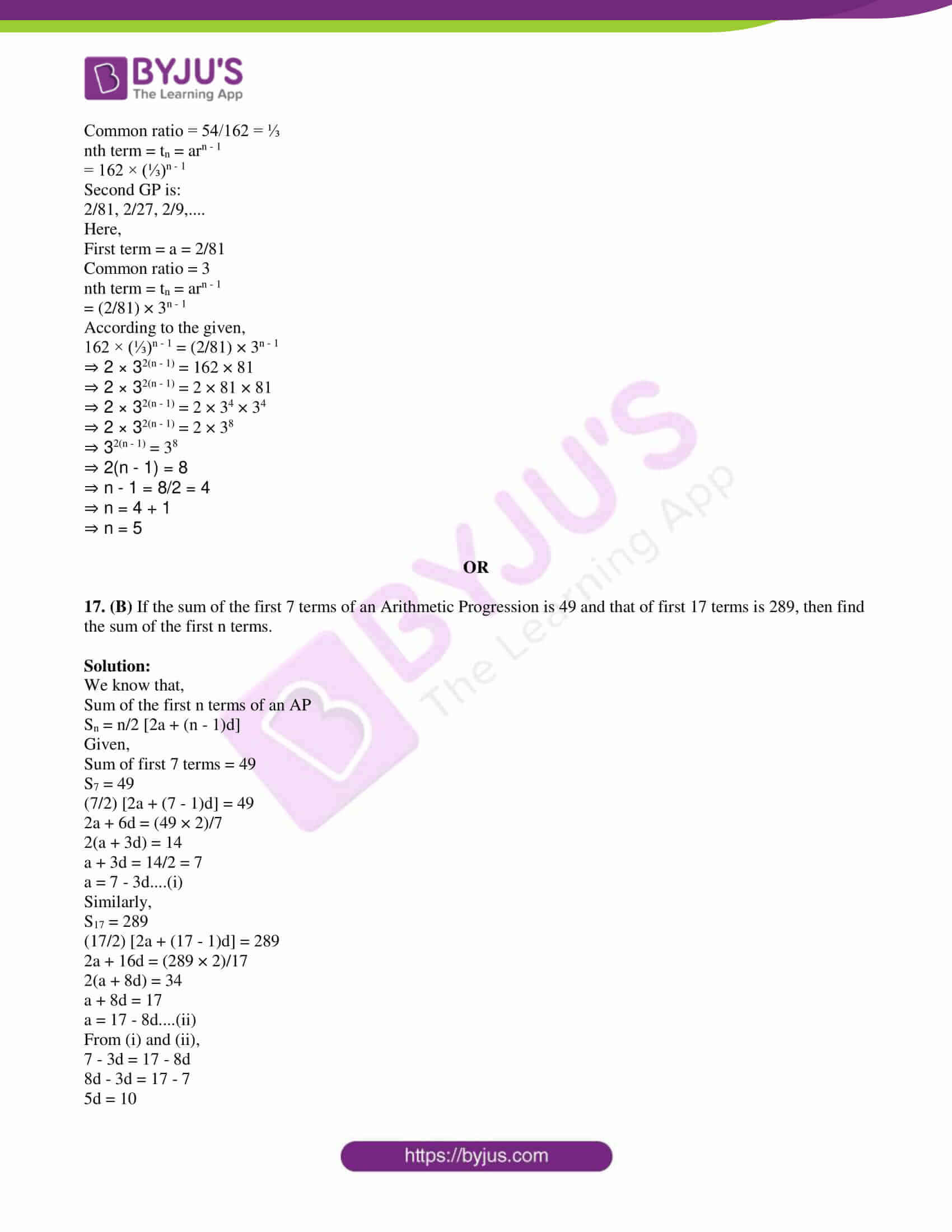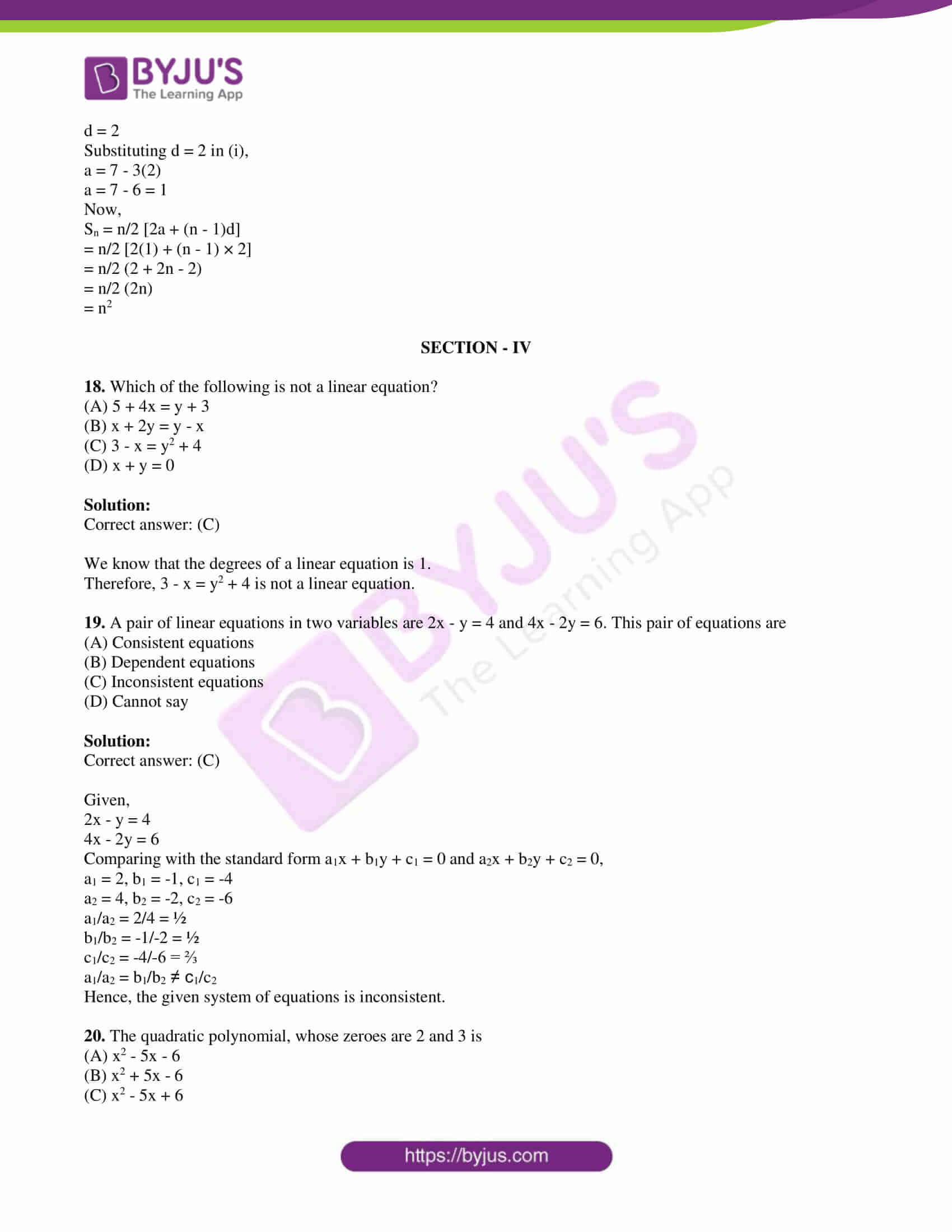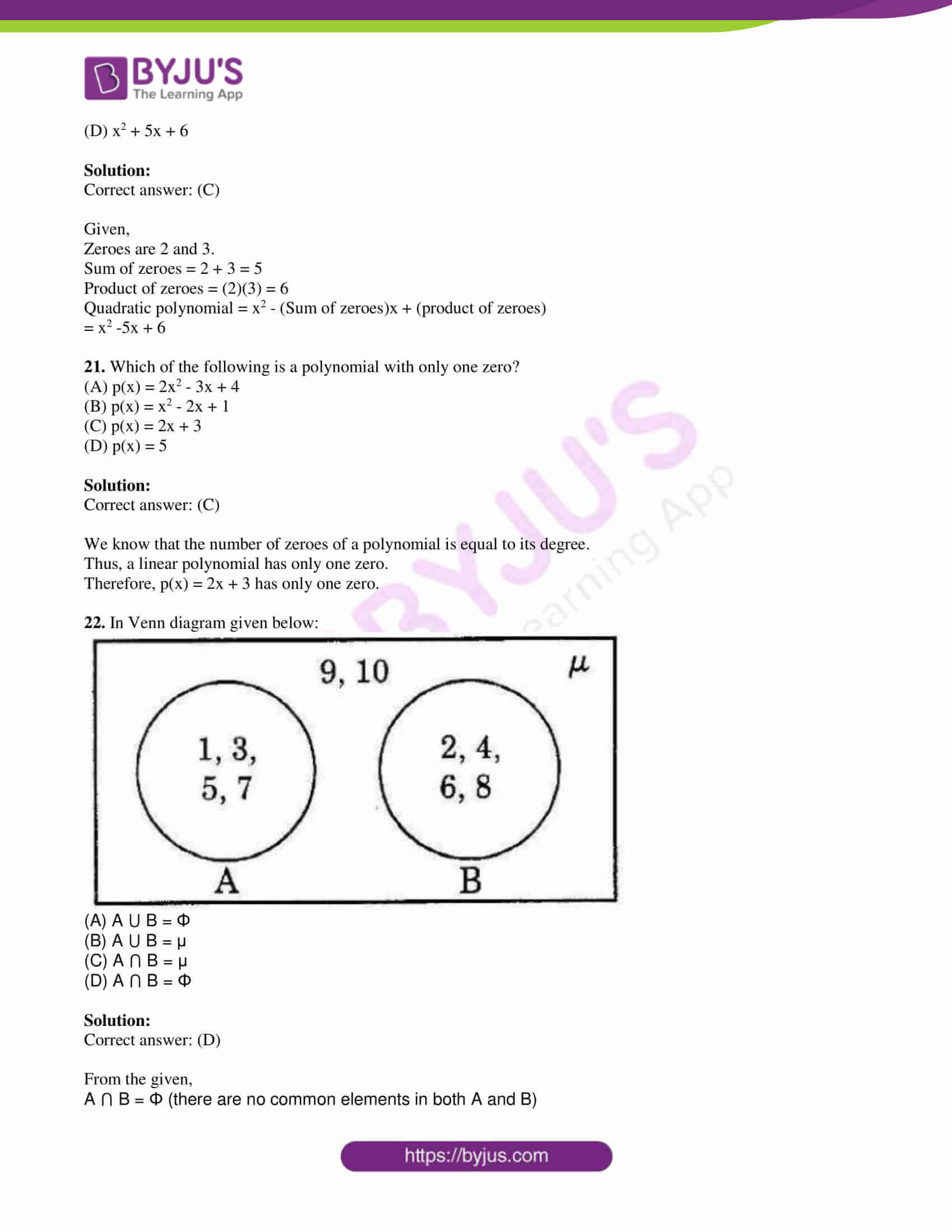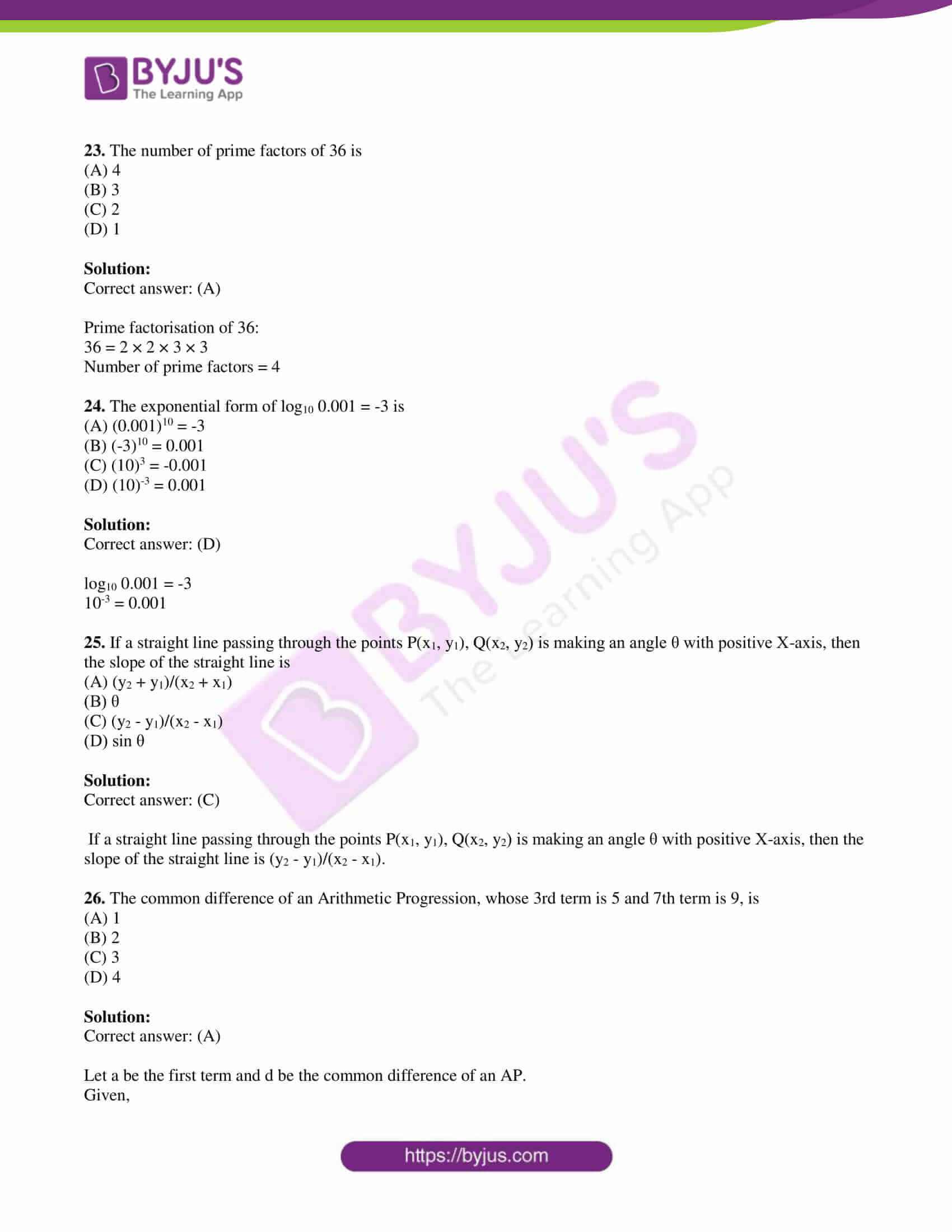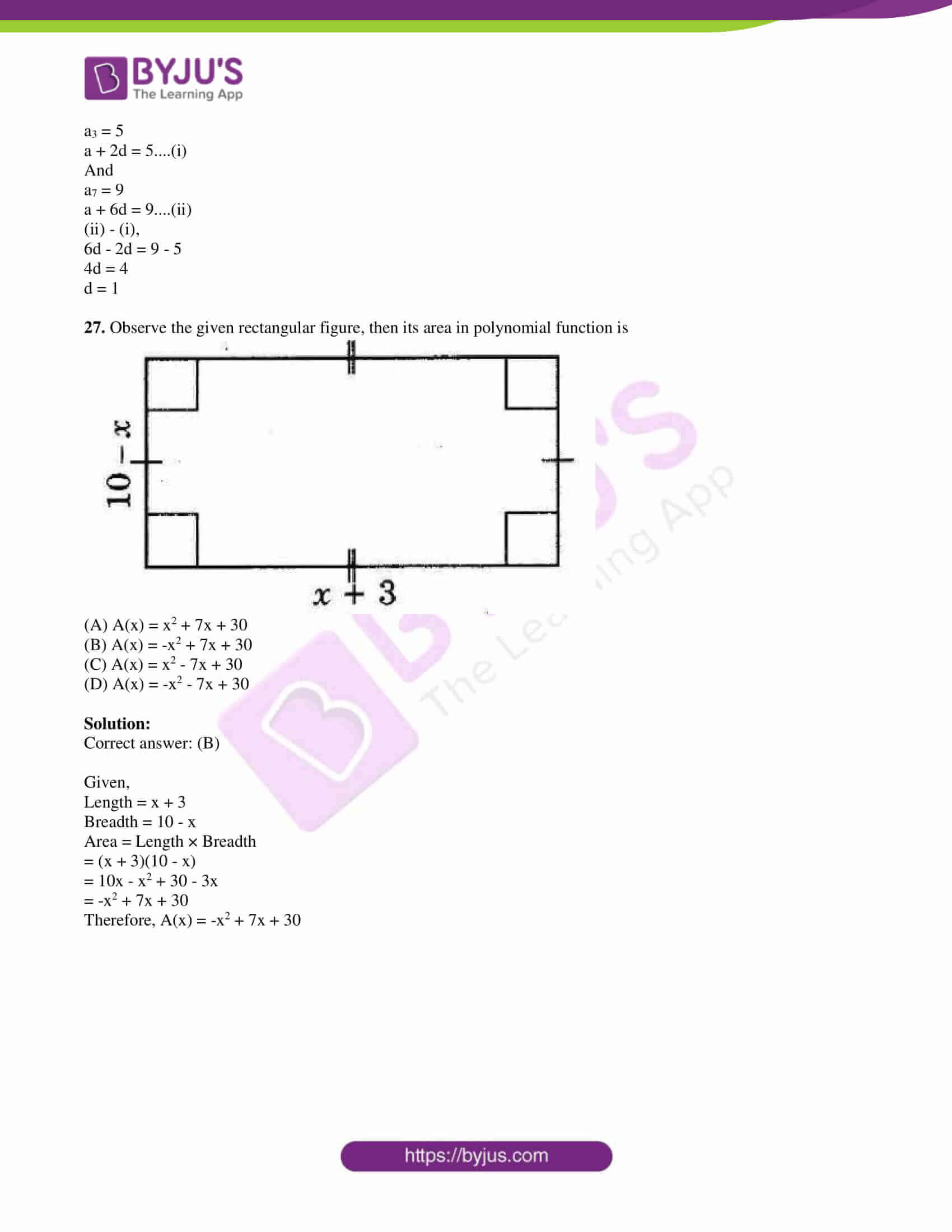PART A

SECTION – I

1. If x ≠ 1, then find the quotient of (x5 + x4 + x3 + x2)/ (x3 + x2 + x + 1).

Solution:

(x5 + x4 + x3 + x2)/ (x3 + x2 + x + 1)

= [x2(x3 + x2 + x + 1)]/ (x3 + x2 + x + 1)

= x2

Hence, the quotient = x2

2. Insert 4 rational numbers between 3/4 and 1 without using (a + b)/2 formula.

Solution:

Let us write 3/4 and 1 with the same denominator.

3/4 = 30/40

1 = 40/40

Hence, 31/40, 32/40, 33/40, 34/40 are the four rational numbers between 3/4 and 1.

3. If A = {x : x ∈ N and x < 20} and B = {x : x ∈ N and x ≤ 5}, then write the set A – B in the set-builder form.

Solution:

Given,

A = {x : x ∈ N and x < 20} = {1, 2, 3, 4, 5, 6, 7, 8, 9, 10, 11, 12, 13, 14, 15, 16, 17, 18, 19}

B = {x : x ∈ N and x ≤ 5} = {1, 2, 3, 4, 5}

A – B = {6, 7, 8, 9, 10, 11, 12, 13, 14, 15, 16, 17, 18, 19}

Set-builder form of A – B = {x : x ∈ N and 5 < x < 20}

4. For what value of K, the following system of equations has a unique solution.

x – Ky = 2

3x + 2y = -5

Solution:

Given,

x – Ky = 2

3x + 2y = -5

Comparing with the standard form a1x + b1y + c1 = 0 and a2x + b2y + c2 = 0,

a1 = 1, b1 = -K, c1 = -2

a2 = 3, b2 = 2, c2 = 5

Condition for unique solution

a1/a2 ≠ b1/b2

1/3 ≠ -K/2

⇒ K ≠ -2/3

Hence, K takes all the real values except -⅔.

5. “We can write a trinomial having degree 7.” Justify the above statement by giving one example.

Solution:

We know that a trinomial has three terms.

The degree of the polynomial is the highest power of the variable in that polynomial.

Therefore, a trinomial with degree 7 is x7 + 2x2 + 5.

6. Find the centroid of a triangle, whose vertices are (3, 4), (-7, -2) and (10, -5).

Solution:

Let the given vertices of a triangle be:

(x1, y1) = (3, 4)

(x2, y2) = (-7, -2)

(x3, y3) = (10, -5)

Centroid of the triangle = [(x1 + x2 + x3)/3, (y1 + y2 + y3)/3]

= [(3 – 7 + 10)/3, (4 – 2 – 5)/3]

= (6/3, -3/3)

= (2, -1)

7. The hand-borewell driller charges Rs. 200/- for the first one metre only and raises drilling charges at the rate of Rs. 30/- for every subsequent metre. Write a progression for the above data.

Solution:

Charge for first metre = Rs. 200

Charge for subsequent metre = Rs. 30

Thus,

For 2 metres = Rs. 230

For 3 metres = Rs. 260

This forms a sequence as: 200. 230, 260, ….

This is an AP with a = 200 and d = 30

SECTION – II

8. Write any three numbers of two digits. Find the LCM and HCF for the above numbers by the “Prime Factorization method”.

Solution:

Let 24, 36 and 58 be the three two digit numbers.

Prime factorisation of 24:

24 = 2 × 2 × 2 × 3

36 = 2 × 2 × 3 × 3

58 = 2 × 29

LCM = 2 × 2 × 2 × 3 × 3 × 29 = 2088

HCF = 2

9. “If we multiply or divide both sides of a linear equation by a non-zero number, then the roots of that linear equation will remain the same”. Is it true? If so, justify with an example.

Solution:

Let 3x = 6 be a linear equation.

The root of this equation is x = 2.

Multiply with 4 on both sides,

12x = 24

x = 2

Divide by 5 on both sides,

3x/5 = 6/5

x = 2

Hence, the given statement is true.

10. In a flower garden, there are 23 plants in the first row, 21 plants in the second row, 19 plants in the third row and so on. If there are 10 rows in that flower garden, then find the total number of plants in the last row with the help of the formula tn = a + (n – 1)d.

Solution:

Given,

Number of plants in the first row = 23

Second row = 21

Third row = 19

Number of rows = 10

Thus, the series is 23, 21, 19,….

This is an AP with a = 23, d = -2 and n = 10

tn = a + (n – 1)d

t10 = 23 + (10 – 1)(-2)

= 23 + (9)(-2)

= 23 – 18

= 5

Hence, the number of plants in the last row = 5

11. Give an example for each of the following:

(i) The product of two irrational numbers is a rational number.

(ii) The product of two irrational numbers is an irrational number.

Solution:

(i) Let √3 and √7 be the two irrational numbers.

Product = √3 × √3 = 3

Thus, the product of two irrational numbers is a rational number.

(ii) Let √2 and √11 be the two irrational numbers.

Product = √2 × √11 = √22

Thus, the product of two irrational numbers is an irrational number.

12. If A = {x : x ∈ N, x < 6} and B = {x : x ∈ N, 3 < x < 8}, then show that A – B ≠ B – A with the help of Venn diagram.

Solution:

Given,

A = {x : x ∈ N, x < 6} = {1, 2, 3, 4, 5}

B = {x : x ∈ N, 3 < x < 8} = {4, 5, 6, 7}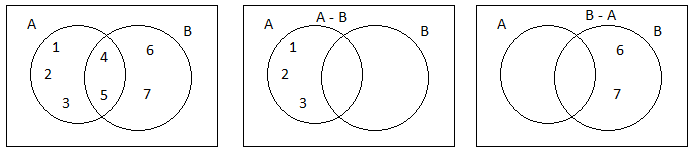Therefore, A – B ≠ B – A

13. Show that the points A(4, 2), B(7, 5) and C(9, 7) are collinear.

Solution:

If (x1, y1), (x2, y2) and (x3, y3) are collinear, then the area of the triangle formed by these vertices is 0.

That means ½ [x1(y2 – y3) + x2(y3 – y1) + x3(y1 – y2)] = 0

Given,

A(4, 2), B(7, 5) and C(9, 7)

Area of triangle ABC = ½ [4(5 – 7) + 7(7 – 2) + 9(2 – 5)]

= ½ [4(-2) + 7(5) + 9(-3)]

= ½ [-8 + 35 – 27]

= ½ (35 – 35)

= 0

Hence, the given points are collinear.

SECTION – III

14. (A) Prove that 3 + 2√5 is an irrational number.

Solution:

Let √3 + 2√5 be a rational number.

3 + 2√5 = a, where a is an integer.

Squaring on both sides,

(3 + 2√5)2 = a2

(3)2 + (2√5)2 + 2(3)(2√5) = a2

9 + 20 + 12√5 = a2

29 + 12√5 = a2

12√5 = a2 – 29

√5 = (a2 – 29)/12

(a2 – 29)/12 is a rational number since a is an integer.

Therefore, √5 is also an integer.

We know that √5 is an irrational number.

Thus, our assumption that 3 + 2√5 is a rational number is wrong.

Hence, 3 + 2√5 is an irrational number.

OR

14. (B) Name the type of the quadrilateral formed by joining the points A(-1, -2), B(1, 0), C(-1, 2) and D(-3, 0) on a graph paper. Justify your answer.

Solution:

Given,

A(-1, -2), B(1, 0), C(-1, 2) and D(-3, 0)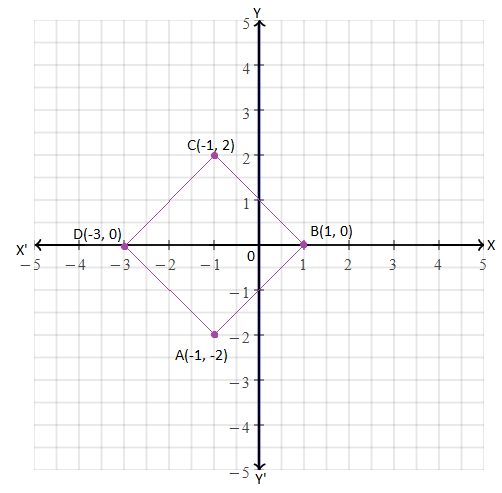From the graph, it is clear that the quadrilateral is a square since all the sides are equal and diagonals are equal.

15. (A) Laxmi does not want to disclose the length, breadth and height of a cuboid of her project. She has constructed a polynomial x3 – 6x2 + 11x – 6 by taking the values of length, breadth and height as its zeroes. Can you open the secret? (i.e. find the measures of length, breadth and height)?

Solution:

Given polynomial is:

x³ – 6x² + 11x – 6

Let us find the three roots of a given equation to get length, breadth and height.

x³ – 6x² + 11x – 6 = 0

x3 – x2 – 5x2 + 5x + 6x – 6 = 0

x2(x – 1) – 5x(x – 1) + 6(x – 1) = 0

(x – 1)(x2 – 5x + 6) = 0

(x – 1)[x2 – 3x – 2x + 6] = 0

(x – 1)[x(x – 3) – 2(x – 3)] = 0

(x – 1)(x – 2)(x – 3) = 0

x = 1, 2, 3

Hence, the length, breadth and height of the cuboid are 3 units, 2 units and 1 unit respectively.

OR

15. (B) Solve the following pair of equations by reducing them to a pair of linear equations.

[5/(x – 1)] + [1/(y – 2)] = 2 and [6/(x – 1)] – [3/(y – 2)] = 1

Solution:

Given,

[5/(x – 1)] + [1/(y – 2)] = 2….(i)

[6/(x – 1)] – [3/(y – 2)] = 1….(ii)

Let 1/(x – 1) = a and 1/(y – 2) = b

From (i),

5a + b = 2….(iii)

From (ii),

6a – 3b = 1….(iv)

(iii) × 3 + (iv),

15a + 3b + 6a – 3b = 6 + 1

21a = 7

a = 7/21

a = ⅓

Substituting a = ⅓ in (ii),

6(⅓) – 3b = 1

3b = 2 – 1

b = ⅓

Now,

1/(x – 1) = ⅓

x – 1 = 3

x = 4

And,

1/(y – 2) = ⅓

y – 2 = 3

y = 5

Hence, the solution of the given pair of equations is x = 4 and y = 5.

16. (A) Draw a graph for the following pair of linear equations in two variables and find their solution from the graph.

2x + y = 5

3x – 2y = 4

Solution:

Given,

2x + y = 5

3x – 2y = 4

Consider the first equation:

2x + y = 5

y = -2x + 5

 x -1 0 1 2 y 7 5 3 1

Now consider another equation:

3x – 2y = 4

2y = 3x – 4

y = (3/2)x – 2

 x -2 0 2 4 y -5 -2 1 4

Graph: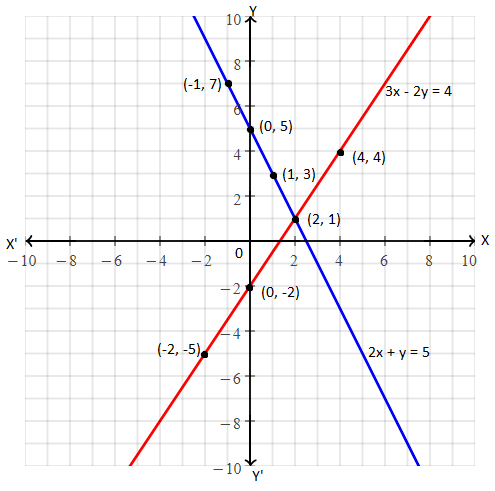The lines representing the given pair of equations intersecting each other at (2, 1).

Hence, the solution of the given pair of linear equations is x = 2 and y = 1.

OR

16. (B) Draw a graph for the polynomial p(x) = x2 + 3x – 4 and find its zeroes from the graph.

Solution:

Given polynomial is:

p(x) = x2 + 3x – 4

Let y = x2 + 3x – 4

 x -5 -4 -2 -1 0 1 2 y 6 0 -6 -6 -4 0 6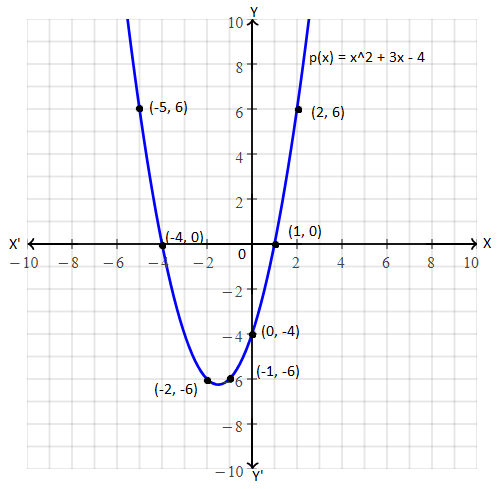The parabola representing the given quadratic polynomial intersecting the x-axis at (-4, 0) and (1, 0).

Hence, the zeroes of the given polynomial are x = -4 and x = 1.

17. (A) If the nth terms of the Geometric progressions 162, 54, 18,…, and 2/81, 2/27, 2/9,…. are equal, then find the value of n.

Solution:

Given,

First GP is:

162, 54, 18,….

Here,

First term = a = 162

Common ratio = 54/162 = ⅓

nth term = tn = arn – 1

= 162 × (⅓)n – 1

Second GP is:

2/81, 2/27, 2/9,….

Here,

First term = a = 2/81

Common ratio = 3

nth term = tn = arn – 1

= (2/81) × 3n – 1

According to the given,

162 × (⅓)n – 1 = (2/81) × 3n – 1

⇒ 2 × 32(n – 1) = 162 × 81

⇒ 2 × 32(n – 1) = 2 × 81 × 81

⇒ 2 × 32(n – 1) = 2 × 34 × 34

⇒ 2 × 32(n – 1) = 2 × 38

⇒ 32(n – 1) = 38

⇒ 2(n – 1) = 8

⇒ n – 1 = 8/2 = 4

⇒ n = 4 + 1

⇒ n = 5

OR

17. (B) If the sum of the first 7 terms of an Arithmetic Progression is 49 and that of first 17 terms is 289, then find the sum of the first n terms.

Solution:

We know that,

Sum of the first n terms of an AP

Sn = n/2 [2a + (n – 1)d]

Given,

Sum of first 7 terms = 49

S7 = 49

(7/2) [2a + (7 – 1)d] = 49

2a + 6d = (49 × 2)/7

2(a + 3d) = 14

a + 3d = 14/2 = 7

a = 7 – 3d….(i)

Similarly,

S17 = 289

(17/2) [2a + (17 – 1)d] = 289

2a + 16d = (289 × 2)/17

2(a + 8d) = 34

a + 8d = 17

a = 17 – 8d….(ii)

From (i) and (ii),

7 – 3d = 17 – 8d

8d – 3d = 17 – 7

5d = 10

d = 2

Substituting d = 2 in (i),

a = 7 – 3(2)

a = 7 – 6 = 1

Now,

Sn = n/2 [2a + (n – 1)d]

= n/2 [2(1) + (n – 1) × 2]

= n/2 (2 + 2n – 2)

= n/2 (2n)

= n2

SECTION – IV

18. Which of the following is not a linear equation?

(A) 5 + 4x = y + 3

(B) x + 2y = y – x

(C) 3 – x = y2 + 4

(D) x + y = 0

Solution:

We know that the degrees of a linear equation is 1.

Therefore, 3 – x = y2 + 4 is not a linear equation.

19. A pair of linear equations in two variables are 2x – y = 4 and 4x – 2y = 6. This pair of equations are

(A) Consistent equations

(B) Dependent equations

(C) Inconsistent equations

(D) Cannot say

Solution:

Given,

2x – y = 4

4x – 2y = 6

Comparing with the standard form a1x + b1y + c1 = 0 and a2x + b2y + c2 = 0,

a1 = 2, b1 = -1, c1 = -4

a2 = 4, b2 = -2, c2 = -6

a1/a2 = 2/4 = ½

b1/b2 = -1/-2 = ½

c1/c2 = -4/-6 = ⅔

a1/a2 = b1/b2 ≠ c1/c2

Hence, the given system of equations is inconsistent.

20. The quadratic polynomial, whose zeroes are 2 and 3 is

(A) x2 – 5x – 6

(B) x2 + 5x – 6

(C) x2 – 5x + 6

(D) x2 + 5x + 6

Solution:

Given,

Zeroes are 2 and 3.

Sum of zeroes = 2 + 3 = 5

Product of zeroes = (2)(3) = 6

Quadratic polynomial = x2 – (Sum of zeroes)x + (product of zeroes)

= x2 -5x + 6

21. Which of the following is a polynomial with only one zero?

(A) p(x) = 2x2 – 3x + 4

(B) p(x) = x2 – 2x + 1

(C) p(x) = 2x + 3

(D) p(x) = 5

Solution:

We know that the number of zeroes of a polynomial is equal to its degree.

Thus, a linear polynomial has only one zero.

Therefore, p(x) = 2x + 3 has only one zero.

22. In Venn diagram given below: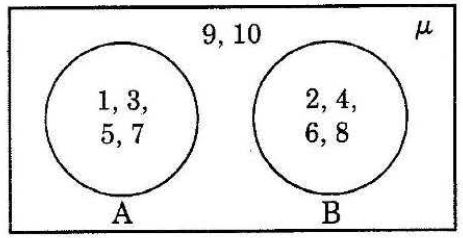(A) A ⋃ B = Φ

(B) A ⋃ B = μ

(C) A ⋂ B = μ

(D) A ⋂ B = Φ

Solution:

From the given,

A ⋂ B = Φ (there are no common elements in both A and B)

23. The number of prime factors of 36 is

(A) 4

(B) 3

(C) 2

(D) 1

Solution:

Prime factorisation of 36:

36 = 2 × 2 × 3 × 3

Number of prime factors = 4

24. The exponential form of log10 0.001 = -3 is

(A) (0.001)10 = -3

(B) (-3)10 = 0.001

(C) (10)3 = -0.001

(D) (10)-3 = 0.001

Solution:

log10 0.001 = -3

10-3 = 0.001

25. If a straight line passing through the points P(x1, y1), Q(x2, y2) is making an angle θ with positive X-axis, then the slope of the straight line is

(A) (y2 + y1)/(x2 + x1)

(B) θ

(C) (y2 – y1)/(x2 – x1)

(D) sin θ

Solution:

If a straight line passing through the points P(x1, y1), Q(x2, y2) is making an angle θ with positive X-axis, then the slope of the straight line is (y2 – y1)/(x2 – x1).

26. The common difference of an Arithmetic Progression, whose 3rd term is 5 and 7th term is 9, is

(A) 1

(B) 2

(C) 3

(D) 4

Solution:

Let a be the first term and d be the common difference of an AP.

Given,

a3 = 5

a + 2d = 5….(i)

And

a7 = 9

a + 6d = 9….(ii)

(ii) – (i),

6d – 2d = 9 – 5

4d = 4

d = 1

27. Observe the given rectangular figure, then its area in polynomial function is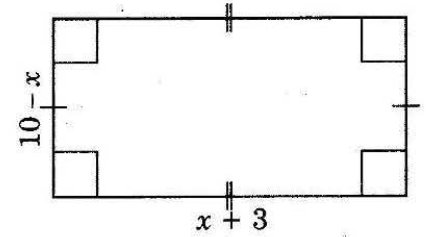(A) A(x) = x2 + 7x + 30

(B) A(x) = -x2 + 7x + 30

(C) A(x) = x2 – 7x + 30

(D) A(x) = -x2 – 7x + 30

Solution:

Given,

Length = x + 3

= (x + 3)(10 – x)

= 10x – x2 + 30 – 3x

= -x2 + 7x + 30

Therefore, A(x) = -x2 + 7x + 30